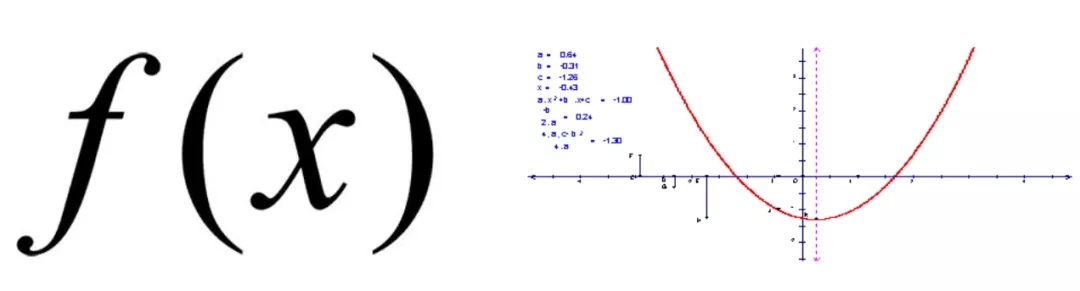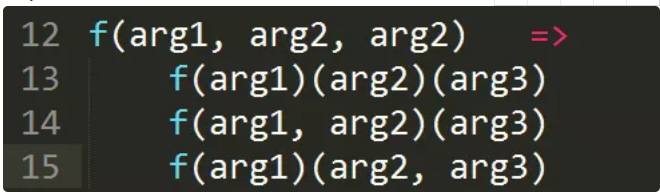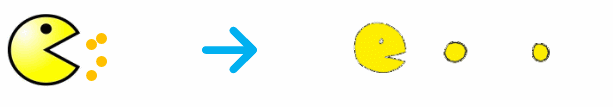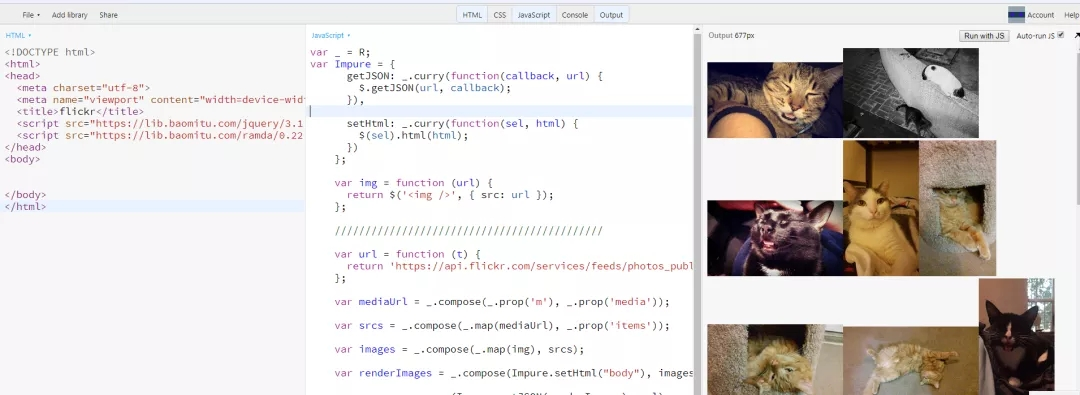### javascript函数式编程基础

[导读] javascript函数式编程基础...

## 三、纯函数（函数式编程的基石，无副作用的函数）```var xs = [1,2,3,4,5];
// 纯的
xs.slice(0,3);
//=> [1,2,3]
xs.slice(0,3);
//=> [1,2,3]
xs.slice(0,3);
//=> [1,2,3]

// 不纯的
xs.splice(0,3);
//=> [1,2,3]
xs.splice(0,3);
//=> [4,5]
xs.splice(0,3);
//=> []

```

```var squareNumber  = memoize(function(x){ return x*x; });
squareNumber(4);
//=> 16
squareNumber(4); // 从缓存中读取输入值为 4 的结果
//=> 16

```

```var minimum = 21;
var checkAge = function(age) {
return age >= minimum;
};

```

```var checkAge = function(age) {
var minimum = 21;
return age >= minimum;
};

```

## 四、函数柯里化

curry 的概念很简单：将一个低阶函数转换为高阶函数的过程就叫柯里化。```//es5写法
return function(y) {
return x + y;
};
};

//es6写法
var add = x => (y => x + y);

//试试看

increment(2);  // 3

```

```var checkage = min => (age => age > min);
var checkage18 = checkage(18);
checkage18(20);
// =>true

```

```var curry = require('lodash').curry;

//柯里化两个纯函数
var match = curry((what, str) => str.match(what));
var filter = curry((f, ary) => ary.filter(f));

//判断字符串里有没有空格
var hasSpaces = match(/\s+/g);

hasSpaces("hello world");  // [ ' ' ]
hasSpaces("spaceless");  // null

var findSpaces = filter(hasSpaces);

findSpaces(["tori_spelling", "tori amos"]);  // ["tori amos"]

```

## 五、函数组合

```var toUpperCase = function(x) { return x.toUpperCase(); };
var exclaim = function(x) { return x + '!'; };

var shout = function(x){
return exclaim(toUpperCase(x));
};

shout("send in the clowns");
//=> "SEND IN THE CLOWNS!"

```

```//定义compose
var compose = (...args) => x => args.reduceRight((value, item) => item(value), x);

var toUpperCase = function(x) { return x.toUpperCase(); };
var exclaim = function(x) { return x + '!'; };

var shout = compose(exclaim, toUpperCase);

shout("send in the clowns");
//=> "SEND IN THE CLOWNS!"
```

```var head = function(x) { return x; };
var reverse = reduce(function(acc, x){ return [x].concat(acc); }, []);

last(['jumpkick', 'roundhouse', 'uppercut']);
//=> 'uppercut'
```

## 六、声明式和命令式代码

```// 命令式
var makes = [];
for (var i = 0; i < cars.length; i++) {
makes.push(cars[i].make);
}

// 声明式
var makes = cars.map(function(car){ return car.make; });
```

## 七、Point Free

pointfree 模式指的是，永远不必说出你的数据。它的意思是说，函数无须提及将要操作的数据是什么样的。一等公民的函数、柯里化（curry）以及组合协作起来非常有助于实现这种模式。

```// 非 pointfree，因为提到了数据：word
var snakeCase = function (word) {
return word.toLowerCase().replace(/\s+/ig, '_');
};

// pointfree
var snakeCase = compose(replace(/\s+/ig, '_'), toLowerCase);
```

## 八、示例应用

1.根据特定搜索关键字构造 url

2.向 flickr 发送 api 请求

3.把返回的 json 转为 html 图片

4.把图片放到屏幕上上面提到了两个不纯的动作，即从 flickr 的 api 获取数据和在屏幕上放置图片这两件事。我们先来定义这两个动作，这样就能隔离它们了。这里我们只是简单包装了一下 jQuery 的 getJSON 函数，把它变为一个 curry 函数，还有就是把参数位置也调换了下，我们把它们放在 Impure 命名空间下以用来隔离，这样我们就知道它们都是危险函数。运用函数柯里化和函数组合的技巧，我们就可以创建一个函数式的实际应用了：## 九、总结